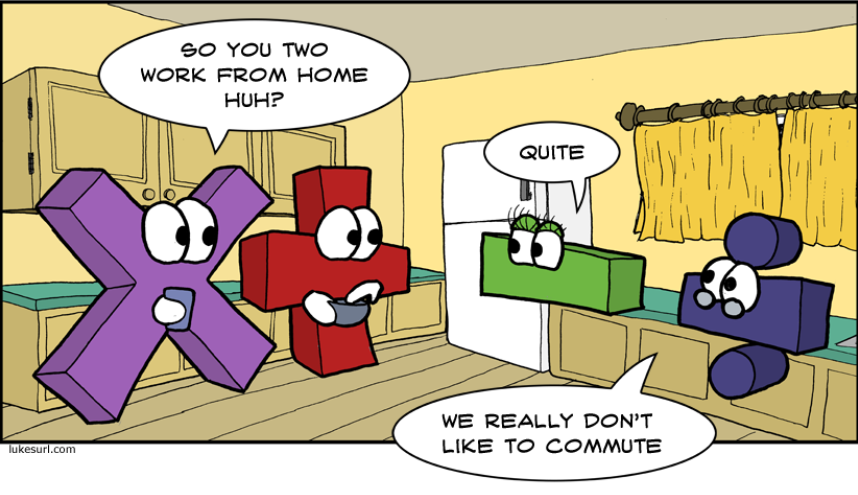## Topic outline

•### General

This is a collection of activities and resources that will help you keep up to date with your maths knowledge and prepare you for the start of your A-Level in maths.

The course is designed for you start at the top of the page and work down through the sections.  Each section should take you approximately 4 hours.

We have tried to pick out topics and thinking skills that are important when you study A-Level maths.

Below we have added a link to collection of weekly resources full of activities including problem solving which have been designed to develop the knowledge and skills you will need for A-Level Maths.•### Level 2 Further Maths

One of the best preparations you can do for A-Level Maths is to be up to date with the Level 2 Further Maths content.

This can be achieved by completing past papers and using Corbett Maths videos and worksheets.

Below is a link to the Corbett Maths webpage, Mrs Walker provided her group with some past papers these are also available at the link below including some video solutions.

•### Trigonometry

Trigonometry is a large proportion of A-Level Maths and it is important you have mastered the topic at GCSE.

Below there are a selection of resources explaining the content you need to know and then worksheets of questions you need to be able to answer.

•### Surds and Indices

A-Level Maths is about precision and Surds are used extensively in calculations and answers.  You need to be able to manipulate them.

A-Level Maths involves a lot of manipulating expressions and you need to be able to interpret and work with indices throughout the course.

Below there are a selection of resources explaining the content you need to know and then worksheets of questions you need to be able to answer.

•### Basic Differentiation

Calculus is a large proportion of the A-Level Maths work you will learn.  You encounter basic differentiation here for the first time, but at A-Level you will develop your skills further and learn the many uses of differentiation.

Below there are a selection of resources explaining the content you need to know and then worksheets of questions you need to be able to answer.

•### Algebraic Fractions

The ability to manipulate and simplify algebraic expressions is essential in all aspects of Pure Mathematics at A-Level.

Below there are a selection of resources explaining the content you need to know and then worksheets of questions you need to be able to answer.

•### Rearranging Formulae

The ability to manipulate and simplify algebraic expressions is essential in all aspects of Pure Mathematics at A-Level.

Below there are a selection of resources explaining the content you need to know and then worksheets of questions you need to be able to answer.

•### Graphs

Graphs allow mathematicians to look at the properties of functions as well as patterns and solutions to problems.

Below there are a selection of resources explaining the content you need to know and then worksheets of questions you need to be able to answer.

•The ability to manipulate and simplify algebraic expressions and solve equations is essential in all aspects of Pure Mathematics at A-Level.

Below there are a selection of resources explaining the content you need to know and then worksheets of questions you need to be able to answer.

•### Circles - Theorems

Understanding the properties of shapes, especially circles is used to explore the properties of graphs at A-Level Maths.

Below there are a selection of resources explaining the content you need to know and then worksheets of questions you need to be able to answer.

•### Circles - Equation

Equations of circles are a part of many aspects of Pure Mathematics.  Their properties are explored further in the A-Level Maths course.

Below there are a selection of resources explaining the content you need to know and then worksheets of questions you need to be able to answer.

•### Proof

The ability to write a reasoned argument and prove general statements is an essential part of mathematics throughout the world.  Without proof we cannot be convinced of the truth of statements.  You will need to be able to write clear, reasoned arguments at A-Level Maths.

Below there are a selection of resources explaining the content you need to know and then worksheets of questions you need to be able to answer.

•### Functions

Functions are used throughout A-level Maths. We use them to model situations and look at their properties.

Below there are a selection of resources explaining the content you need to know and then worksheets of questions you need to be able to answer.